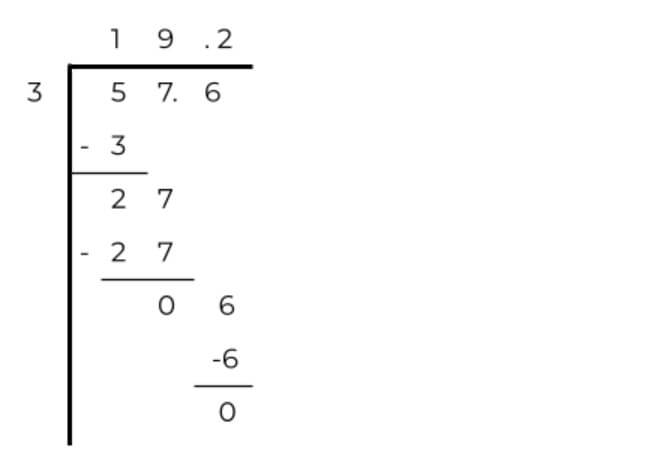# Decimal Calculator

Type the decimal numbers in the decimals calculator to perform various arithmetic operations.## Decimal Calculator

Decimal calculator with steps performs the basic mathematical operations on the numbers, specifically decimal numbers.

The formula and step-by-step calculation display allow the user to understand how the answer was computed, proving it to be a trustable tool.

From essential functions like adding and multiplying to a little complex operation like the logarithm of decimal numbers, this calculator can do it all.

## How to use this tool?

The easy and understandable interface makes it convenient to use. You will have to:

1. Enter the decimal numbers.
2. Choose the operation.
3. Hit the Calculate button.

## What are decimal numbers?

The decimal numbers are a mix of fractions and whole numbers. The whole number part is separated from the fraction by the decimal point.

An example of a decimal number is 98.9. The 98 on the left side is the whole number. Then comes the decimal point and at the end is the 9 for the fraction which actually means 9/10.

You can convert any fraction to decimal using the fraction to decimals calculator

## Arithmetic operations on decimal numbers

For arithmetic operations like dividing or subtracting, try the calculator above. You will not only get the result but also the steps which can be of great help to users who want to learn more about decimal numbers.

Stay tuned to learn through examples.

### How to add or subtract decimal numbers?

The technique of adding or subtracting decimal numbers is easy. Use the calculator, just kidding ????. Simply add the whole numbers to whole numbers and the fraction part to the other fraction part.

Example:

Add the following numbers: 561.1 and 234.9.

Solution:

Step 1: Write the numbers above each other.

5 6 1 . 1

+ 2 3 4 . 9

__________

5 6 1 . 1

+ 2 3 4 . 9

__________

7 9 6 . 0

An example of subtraction using the same numbers.

After writing the numbers, start subtracting. Take one carry from the left to the right. The 1 on the right will become 11.

5 6 1 . 1

- 2 3 4 . 9

__________

3 2 6 . 2

### How to divide or multiply the decimal numbers?

The multiplication or division techniques of decimals are a little different from whole numbers. In multiplying, the only difference comes in placing the decimal point.

For clarity, see the example ahead.

Example:

Multiply 67.3 by 7.1.

Solution:

Step 1: Multiply the numbers.

6 7 . 3

x 7 . 1

_________

6 7 3

4 7 1 1 x

_________
4 7 7 8 3

Step 2: Count the digits after decimal points in both numbers collectively and place the decimal point after counting the same amount of digits from the right.

There are 2 digits so the point will be placed after 2 digits from the right like 477.83.

In dividing the decimal numbers, you have to see if the divisor is a whole number or not. It must be a whole number to carry out the division.

Example:

Divide 5.76 by 0.3

Solution:

Step 1: Convert the decimal to the whole number.

If we want to change the divisor, the same changes will be applied to the dividend.

Multiply both values by 10.

5.76 x 10 = 57.6 , 0.3 x 10 = 3

Step 2: Now divide.Hence; 5.76/0.3 = 19.2

These were the basic four operations. Hope this helps.